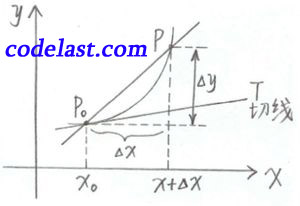## [原创]高等数学笔记(23)

【前言】

【正文】
<定义2> 设函数 $f(x)$${x_0}$ 点的左侧 $[{x_0} + \Delta x,{x_0}]$$\Delta x < 0$ ）有定义，如果极限 $\mathop {\lim }\limits_{\Delta x \to {0^ - }} \frac{{f({x_0} + \Delta x) - f({x_0})}}{{\Delta x}}$ 存在，则称此极限为 $f(x)$${x_0}$ 点的左导数，记为 ${{f'}_ - }({x_0}) = \mathop {\lim }\limits_{\Delta x \to {0^ - }} \frac{{f({x_0} + \Delta x) - f({x_0})}}{{\Delta x}}$

${{f'}_ + }({x_0}) = \mathop {\lim }\limits_{\Delta x \to {0^ + }} \frac{{f({x_0} + \Delta x) - f({x_0})}}{{\Delta x}}$

${f({x_0})}$${{x_0}}$ 点可导 $\Leftrightarrow {{f'}_ - }({x_0}),{{f'}_ + }({x_0})$ 存在且 ${{f'}_ - }({x_0}) = {{f'}_ + }({x_0})$

$f'({x_0}) = \mathop {\lim }\limits_{\Delta x \to 0} \frac{{\Delta y}}{{\Delta x}}$

$\mathop {\lim }\limits_{\Delta x \to 0} \frac{{\Delta y}}{{\Delta x}} = f'({x_0})$$f'(x)$ 在几何上表示曲线上一点 ${P_0}({x_0},f({x_0}))$ 点处切线 ${P_0}T$ 的斜率 $f'({x_0}) = \tan \alpha$$\alpha$ 是切线 ${P_0}T$ 的倾角。

$y = f(x)$${x_0}$ 点处 $f'({x_0}) = \infty$ （表示切线垂直于 $x$ 轴），则切线方程为 $x = {x_0}$

<定理> 如果函数 $y = f(x)$${x_0}$ 点可导，则 $f({x_0})$${x_0}$ 点必定连续。

$\frac{{\Delta y}}{{\Delta x}} = f'({x_0}) + \alpha$

$\mathop {\lim }\limits_{\Delta x \to 0} \Delta y = f'({x_0})\mathop {\lim }\limits_{\Delta x \to 0} \Delta x + \mathop {\lim }\limits_{\Delta x \to 0} (\alpha \Delta x)$
（注： $\alpha \Delta x$ 为两个无穷小的乘积，仍为无穷小）

（第23课完）

➤➤ 版权声明 ➤➤